# Subject 3. Investment Decision Criteria

#analyst-notes #cfa-level-1 #corporate-finance #has-images #reading-35-capital-budgeting #study-session-10

When a firm is embarking upon a project, it needs tools to assist in making the decision of whether to invest in the project or not. In order to demonstrate the use of these four methods, the cash flows presented below will be used.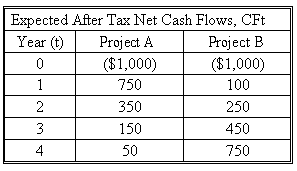Net Present Value (NPV)

This method discounts all cash flows (including both inflows and outflows) at the project's cost of capital and then sums those cash flows. The project is accepted if the NPV is positive.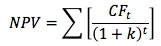where CFt is the expected cash flow at period t, k is the project's cost of capital, and n is its life.

• Cash outflows are treated as negative cash flows since they represent expenditures of the company to fund the project.
• Cash inflows are treated as positive cash flows since they represent money being brought into the company.

The NPV represents the amount of present-value cash flows that a project can generate after repaying the invested capital (project cost) and the required rate of return on that capital. An NPV of zero signifies that the project's cash flows are just sufficient to repay the invested capital and to provide the required rate of return on that capital. If a firm takes on a project with a positive NPV, the position of the stockholders is improved.

Decision rules:

• The higher the NPV, the better.
• Reject if NPV is less than or equal to 0.

NPV measures the dollar benefit of the project to shareholders. However, it does not measure the rate of return of the project, and thus cannot provide "safety margin" information. Safety margin refers to how much the project return could fall in percentage terms before the invested capital is at risk.

Assuming the cost of capital for the firm is 10%, calculate each cash flow by dividing the cash flow by (1 + k)t where k is the cost of capital and t is the year number. Calculate the NPV for Project A and B above.

NPV = CF0 + CF1 + CF2 + CF3 + CF4

Project A's NPV = -1,000 + 750/1.101 + 350/1.102 + 150/1.103 + 50/1.104 = -1,000 + 682 + 289 + 113 + 34 = $118 (rounded) Project B's NPV = -1,000 + 100/1.101 + 250/1.102 + 450/1.103 + 750/1.104 = -1,000 + 91 + 207 + 338 + 512 =$148 (rounded)

Internal Rate of Return (IRR)

This is the discount rate that forces a project's NPV to equal zero.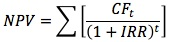Note that this formula is simply the NPV formula solved for the particular discount rate that forces the NPV to equal zero. The IRR on a project is its expected rate of return. The NPV and IRR methods will usually lead to the same accept or reject decisions.

Decision rules:

• The higher the IRR, the better.
• Define the hurdle rate, which typically is the cost of capital.
• Reject if IRR is less than or equal to the hurdle rate.

IRR does provide "safety margin" information.

Calculate Project A's and B's IRR.

Project A: -1000 + 750/(1 + IRR)1 + 350/(1+IRR)2 + 150/(1+IRR)3 + 50/(1+IRR)4 = 0

Since it is difficult to determine by hand, the use of a financial calculator is needed to solve for IRR.

The IRR for Project A is 18.32% and for Project B is 15.03%.

Payback Period

This is the expected number of years required to recover the original investment. Payback occurs when the cumulative net cash flow equals 0.

Decision rules:

• The shorter the payback period, the better.
• A firm should establish a benchmark payback period. Reject if payback is greater than benchmark.

PaybackA = 1 + (1000 - 750)/350 = 1.7 years
PaybackB = 3 + (1000 - 100 - 250 - 450)/750 = 3.27 years

Drawbacks:

• It ignores cash flows beyond the payback period. Payback period is a type of "break-even" analysis: it indicates how quickly you can make enough money to recover the initial investment, not how much money you can make during the life of the project.
• It does not consider the time value of money. Therefore, the cost of capital is not reflected in the cash flows or calculations.

Discounted Payback Period

This is similar to the regular payback method except that it discounts cash flows at the project's cost of capital. It considers the time value of money, but it ignores cash flows beyond the payback period.

Again, assume the cost of capital for the firm is 10%:
Discounted PaybackA = 2 + (1000 - 682 - 289)/113 = 2.26 years
Discounted PaybackB = 3 + (1000 - 91 - 207 - 338)/512 = 3.71 years

The payback provides an indication of a project's risk and liquidity because it shows how long the invested capital will be tied up in a project and "at risk." The shorter the payback period, the greater the project's liquidity, the lower the risk, and the better the project. The payback is often used as one indicator of a project's risk.

Average Accounting Rate of Return
(not required)

This is a very simple rate of return: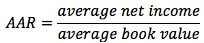Its only advantage is that it is very easy to calculate.

Drawbacks:

• It does not take into account the time value of money; the value of cash flows does not diminish with time, as is the case with NPV and IRR.
• ARR is based on numbers that include non-cash items.

Profitability Index (PI)

This is an index used to evaluate proposals for which net present values have been determined. The profitability index is determined by dividing the present value of each proposal by its initial investment.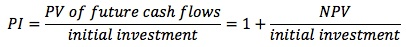The PI indicates the value you are receiving in exchange for one unit of currency invested.

• An index value greater than 1.0 is acceptable and the higher the number, the more financially attractive the proposal.
• A ratio of 1.0 is logically the lowest acceptable measure on the index. Any value lower than 1.0 would indicate that the project's PV is less than the initial investment.

Learning Outcome Statements

d. calculate and interpret net present value (NPV), internal rate of return (IRR), payback period, discounted payback period, and profitability index (PI) of a single capital project;